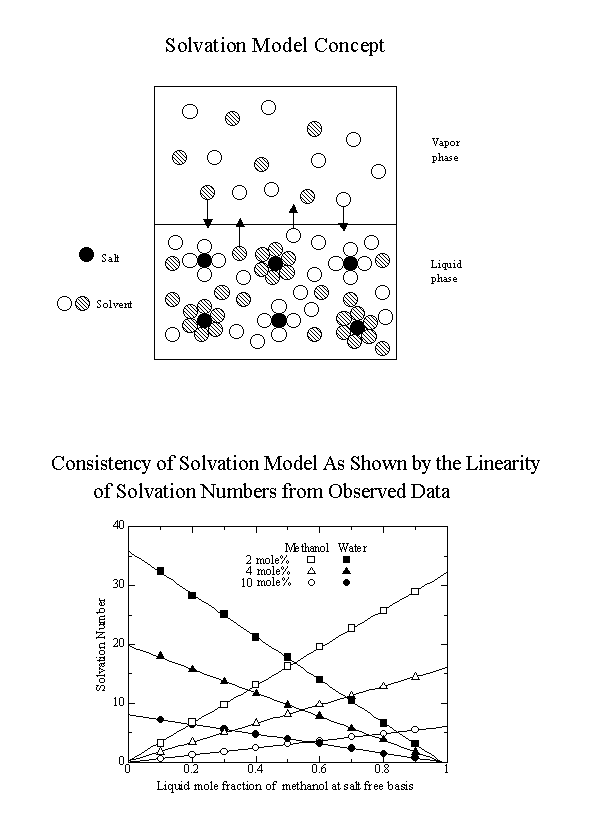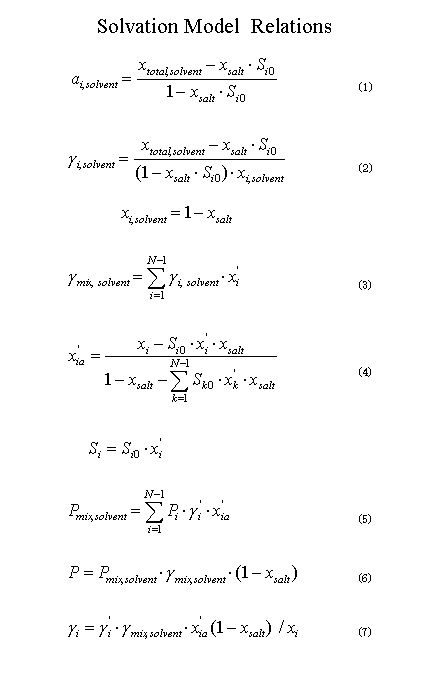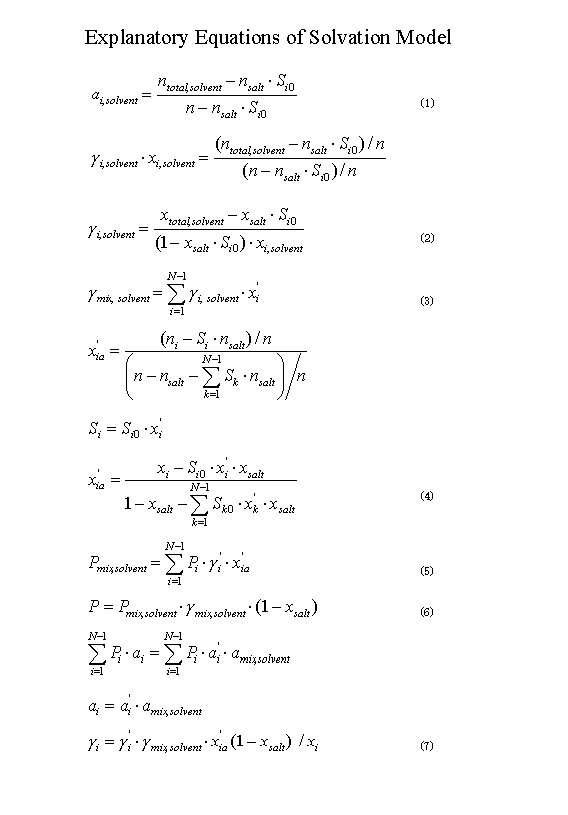ü@

Vapor-Liquid Equilibria by the Solvation Method

Shuzo Ohe, Ph.D. Prof. Sci. Univ. Tokyoü@ü@ü@
 üyPrediction Exampleüz Calculate vapor-liquid equilibria for the methanol water CaCl2 (4 mole %) system at 1 atm by solvation method when the liquid composition of methanol is 60 mole % at salt free basis and the solvation number of CaCl2 to methanol is 15.395 and that to water is 18.7844. üySolutionüz From the given liquid compositions xi'üü 0.6, xsaltüü 0.04, xtotal,solventüü 0.96 then compositions at salt basis are calculated as xéP= 0.6üE0.96 = 0.576, x2= 0.4üE0.96 = 0.384, respectively. The solvation number for each volatile component is given as ü@ü@ü@ü@ü@S10 = 15.395,ü@ü@S20 = 18.7844 therefore, from relation; ü@Si = Sioü~xi'ü@they are estimated as S1= 15.395ź0.6 = 9.237, S2= 18.7844ź0.4 = 7.514, respectively. Next, effective liquid compositions of each volatile component from Eq. (4) is, calculated as x1a'ü@= (0.576 - 9.237ź0.04) / (1- 0.04 - 9.237ź0.04 - 7.514ź0.04) ü@ü@ü@= 0.7122ü@ Similarly,ü@x2a' = 0.2878. ü@ Activity coefficients g1' and g2'ü@for effective liquid compositions: x1a', x2a' are calculated by the Wilson equation, using the following parameters for the methanol water system: ü@ü@ü@ā®12 = 0.5515 and ā®2éP = 0.8978. By applying the Wilson equation: ü@ ln g1'ü@= -ln (x1a' ā®12 x2a') x2a' [ā®12 / ( x1a' ā®12 x2a') -ā®2éP / (ā®2éPx1a' x2a')] ü@ ln g2'ü@= -ln (x2a' ā®2éP x1a') - x1a' [ā®12 / ( x1a' ā®12 x2a') -ā®2éP / (ā®2éPx1a' x2a')] to the compositions x1a' and x2a' , we get ln g1'= 0.0418, ln g2' = 0.3142 then g1' = 1.0427, g 2' = 1.3692. ü@ Second, determine the activity coefficient for vapor pressure loweringü@gmix,solvent . From the solvation number for each pure solvent S10, S20, Eq. (2) estimates activity coefficients for respective components: g 1,solventü@and g2, solvent. g 1,solventü@= (0.96 - 0.04üE15.395) / (1 - 0.04üE15.395) / 0.96ü@= 0.9332 g2, solvent = (0.96 - 0.04üE15.395) / (1- 0.04üE18.7844) / 0.96ü@= 0.8741 Eq. (3) calculates activity coefficients of solvent mixture g mix, solvent. ü@ g mix, solvent = 0.9332ź0.6 0.8741ź0.4 = 0.9096 Total activity coefficients for each volatile component are calculated from Eq. (7) asü@ ü@ g1ü@= 1.0427üE0.9096üE0.7122üE0.96 / 0.576 = 1.1257 ü@ g2ü@= 1.3692üE0.9096üE0.2878üE0.96 / 0.384 = 0.8961. ü@ Calculate the vapor pressures for each volatile component from the Antoine equation. Antoine constants for methanol and water are A1ü@= 8.07919üAB1ü@= 1581.341üAC1ü@= 239.65 A2ü@= 8.02754üAB2ü@= 1705.616üAC2ü@= 231.405. The Equilibrium temperature can be determined by bubble point calculation as 72.58 üÄ. Then the methanol vapor pressure is P1 = 10 ( 8.07919 - 1581.341 / (72.58 239.65) ) = 1034.01 mmHg and that of water is P2 = 10 ( 8.02754 - 1705.616 / (72.58 231.405) ) = 261.03 mmHg. Therefore, methanol and water partial pressures are p1 = 1034.01üE1.1257üE0.576 = 670.46 mmHg p2 = 261.03üE0.8961üE0.384 = 89.82 mmHg. The total pressure is then ü@ā╬ = p1 p2 = 760.28 mmHg. Vapor compositions for the components y1, y2 are y1 = p1 /ā╬ = 670.46 / 760.28 = 0.882, y2 = 0.118. The observed bubbling point is 72.6 üÄüAand the vapor phase composition of methanol is 0.884 mole fraction. Absolute errors are 0.02 üÄ and 0.002, which indicate a high degree of accuracy.